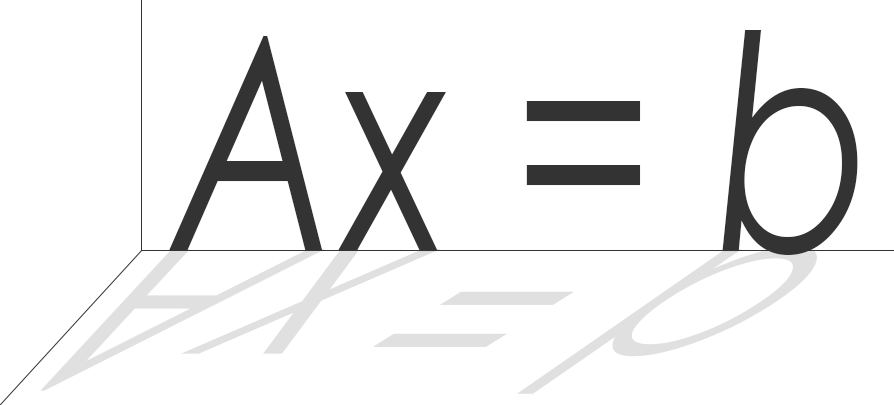# linear-algebra-js

View the Project on GitHub

linear-algebra-js is an optimized linear algebra library in pure Javascript. It supports both sparse and dense matrices, with routines to compute Cholesky, LU, and QR factorizations.

linear-algebra-js is implemented in asm.js to obtain the best possible performance for a Javascript program, and was compiled from a wrapper around the C++ library Eigen with emscripten. Future updates will compile the more optimized sparse matrix library Suitesparse to asm.js.

Detailed documentation and unit tests can be found in the docs and tests directories of this repository.

## Code Snippet

``````// solve the linear system Ax = b, where A is a square sparse matrix
let A = SparseMatrix.identity(5, 5);
let b = DenseMatrix.ones(5, 1);

let lu = A.lu();
let x = lu.solveSquare(b);

b.scaleBy(5);
x = lu.solveSquare(b); // factorization is reused
``````

## Getting started

1. Clone this repository
``````git clone https://github.com/rohan-sawhney/linear-algebra-js.git
``````
2. Include the files in the src directory in your browser or node js code.

Rohan Sawhney